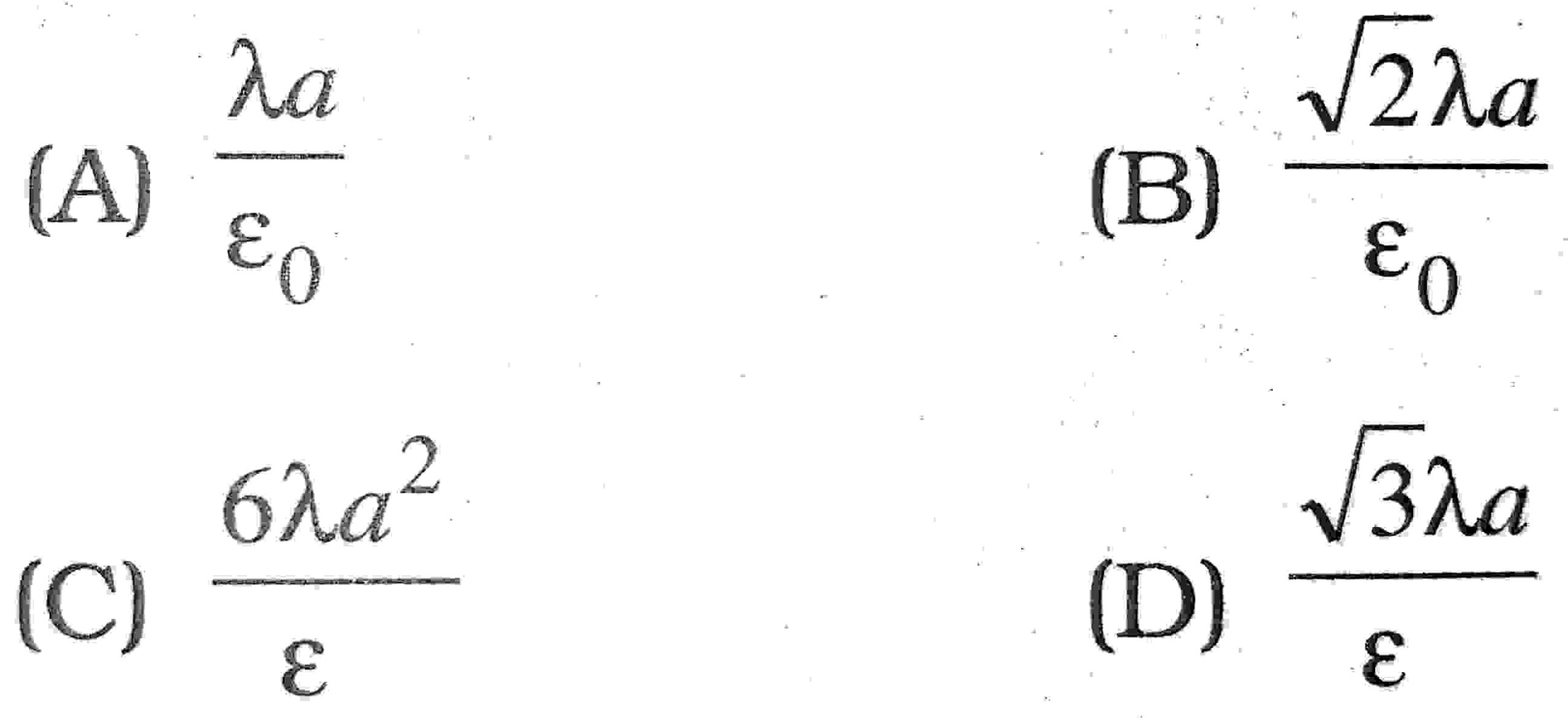1. Which one of the following is the unit of electric field ?

(A) Coulomb
(B) Newton
(C) Volt
(D) N/C

2. If an electric dipole is kept in a uniform electric field then resultant electric force on it is :

(A) always zero
(B) never zero
(C) depend upon capacity of dipole
(D) None

3. The number of electron taken out from a body to produce 1 coulomb of charge will be:

(A) 6.25 x 1018
(B) 6.25 x 108
(C) 6.023 x 1023
(D) None

4. The work done in rotating an electric dipole in an electric field :

(A) W = ME (1 – cosθ)
(B) W = ME tanθ
(C) W = ME secθ
(D) None

5. If sphere of bad conductor is given charge then it is distributed on :

(A) surface
(B) inside the surface
(C) only inside the surface
(D) None

6. Electric field in a cavity of metal :

(A) depends upon the surroundings
(B) depends upon the size of cavity
(C) is always zero
(D) is not necessarily zero

7. The dielectric constant of a metal is :

(A) O
(B) 1
(C) ∞
(D) -1

8. I coulomb is equal to :

(A) 3 x 109 e.s.u.
(B) 1/3 x 109 e.s.u.
(C) 3 x 1010 e.s.u.
(D) 1/3 x 1010 e.s.u.

9. Each of the two point charges are doubled and their distance is halved. Force 77 interaction becomes p times, where p is :

(A) 1
(B) 4
(C) 1/16
(D) 16

10. Coulomb’s law in vector form can be written as :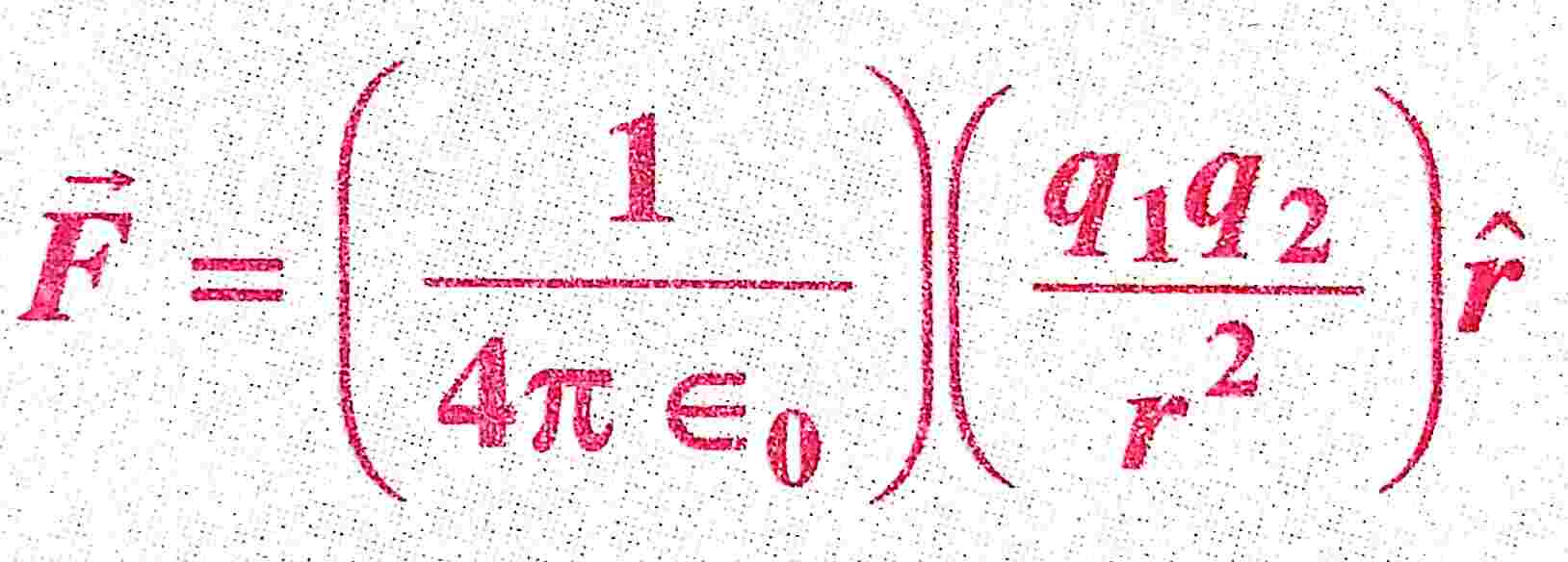where ε0 is the permitivity of free space. The SI units of ε0 will be :

(A) N-1 m-2 C-2
(B) N m-2 C2
(C) N-1 m-2 C2
(D) N m-2 C2

11. The dimensional representation of e० will be:

(A) [MLT4 A2]
(B) [M-1 L-3 T4 A2]
(C) [ML-2 T2 A-2]
(D) None of these

12. When placed in a uniform field, a dipole experiences :

(A) a net force
(B) a torque
(C) both a net force and torque
(D) neither a net force nor a torque

13. A parrot comes and sits on a bare high power line. It will :

(A) experience a mild shock.
(B) experience a strong shock.
(C) get killed instantaneously.
(D) not be affected practically.

14. The SI units of electric dipole moment are :

(A) C
(B) Cm-1
(C) Cm
(D) Nm-1

15. If two conducting sphere are connected after charging separately then :

(A) Electrostatic energy sphere energy wil remain conserved
(B) Electrostatic energy charges remains conserved
(C) Electrostatic energy energy and charge remains conserved
(D) None

16. A semicircular arc of radius r is charged uniformly and the charge per unit length is λ The electric field at the centre is :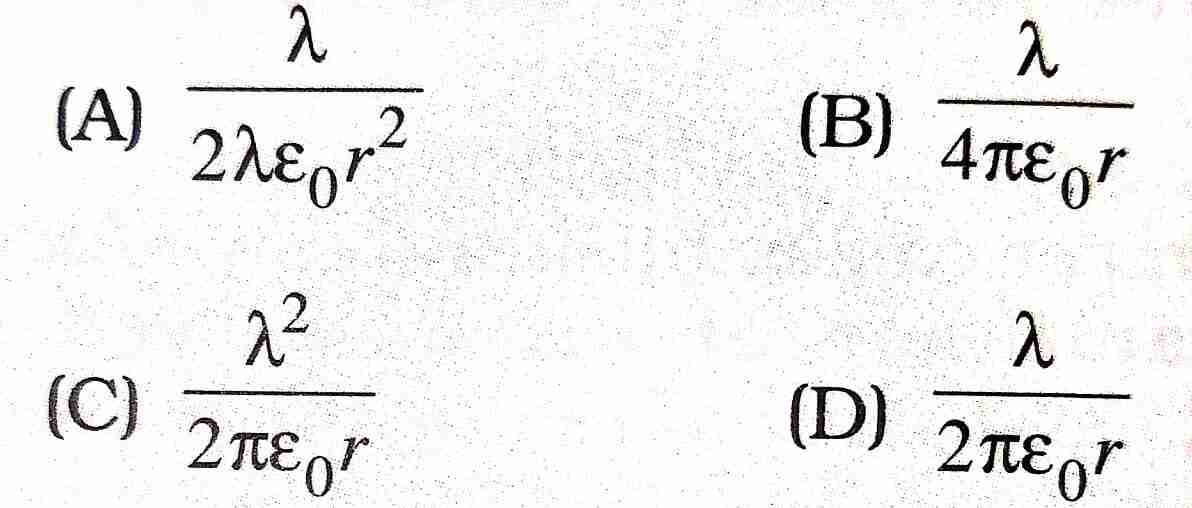17. When a dipole of moment ” p is placed in uniform electric field e, then the torque acting on the dipole is :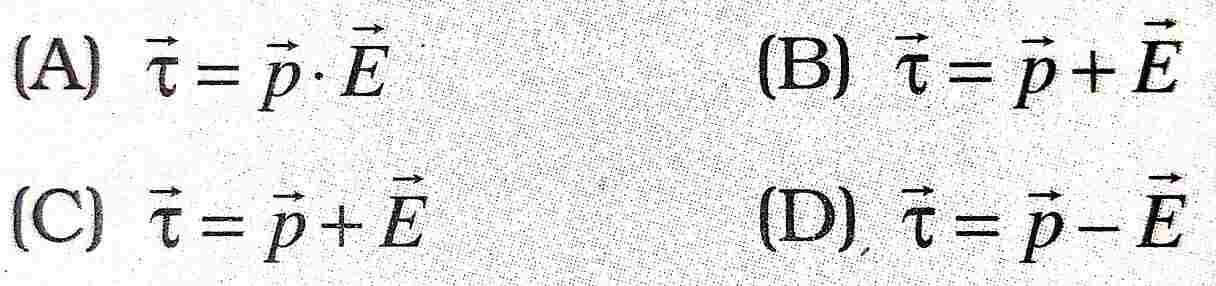18. The graph drawn between V are r for a non-conducting charged solid sphere of radius R for r<R will be :

(A) straight line
(B) parabola
(C) hyperbola
(D) None of these

19. Corona discharge takes place :

(A) at the surface of a conductor
(B) near the sharp points of a conductor
(C) outside the conductor
(D) at the centre of the conductor

20. The belt of a Vande Graaf generator gets charge by :

(A) corona discharge
(B) induction
(C) electrification
(D) None of these

21. Quantisation of charge implies :

(A) Charge does not exist
(B) Charge exists on particles
(C) There is a minimum permissible magnitude of charge
(D) Charge can’t be created

22. The conservation of electric charge implies that :

(A) Charge can’t be created
(B) Charge can’t be destroyed
(C) The number of charged particle in the universe is constant
(D) Simultaneous creation of equal and opposite charges is permissible

23. The number of electrons contained in one coulomb of charge is :

(A) 6.25 x 1018
(B) 6.25 x 1017
(C) 6.25 x 1019
(D) 1.6 x 1019

24. The unit of intensity of electric field is :

(A) metre/volt
(B) Joule/newton
(C) Coulomb/newton
(D) Newton/coulomb

25. 1 V equals to :

(A) 1 J
(C) 1 JC-1
(C) 1 CJ-1
(D) 1 JC

26. 1 Vm-1 equals to :

(A) 1 N
(B) 1 Mm-1
(C) 1 NC-1
(D) 1 J-1

27. The minimum amount of charge observed so far is :

(A) 1 C
(B) 4.8 x 1-13 C
(C) 1.6 × 10-19 C
(D) 1.6 x 1019 C

28. The ratio of electric force between two electrons to the gravitational force between them is of the order :

(A) 1042
(B) 1039
(C) 1036
(D) 1

29. A spherical equipotential surface is not possible :

(A) for a point charge
(B) for a dipole
(C) inside a uniformly charged sphere
(D) inside a spherical conductor

30. Charge on one proton in Coulomb is :

(A) 1.6 x 10-19
(B) 9.1 x 10-31
(C) – 1.6 x 10-19
(D) None of these

31. The energy density at a point in vacuum where the electric field is E, will be :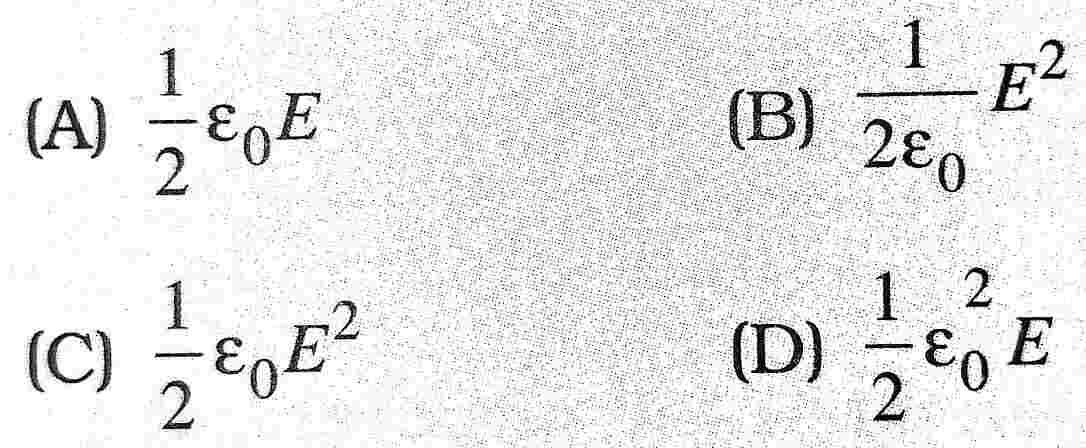32. Electric field intensity at the centre of an electric dipole of length 2d is :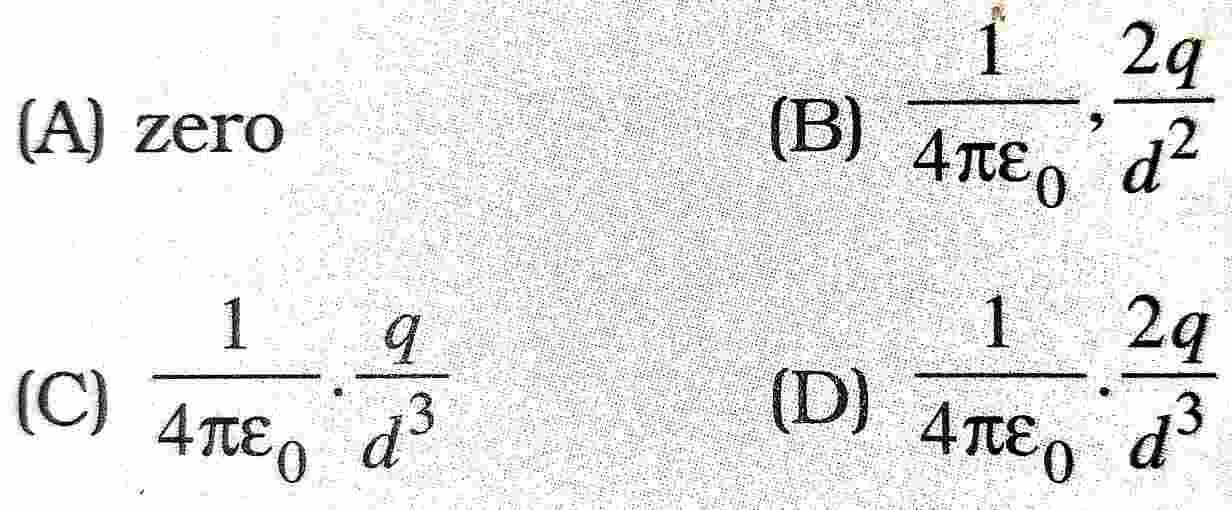33. Electric field due to a solid non-conducting sphere inside varies as :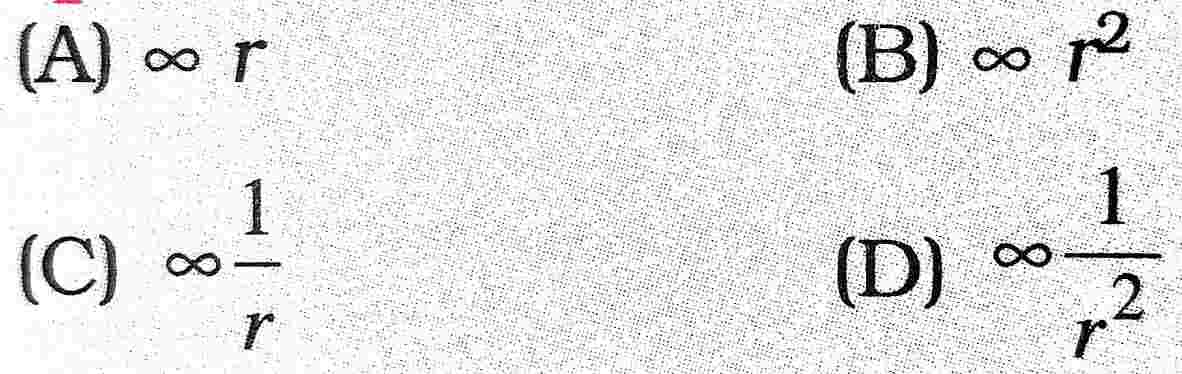34. Ratio of electric fields due to an electric dipole on its axis and on the perpendicular bisector of the dipole at the same distance is :

(A) 4:1
(B) 1:4
(C) 2:1
(D) 1:2

35. SI unit of dielectric constant is :

(A) NM-2 C-2
(B) NM-2 C2
(C) No unit
(D) F/N

36. A point charge +q is placed at the mid-point of a cube of side a. The electric flux emerging from a cube is :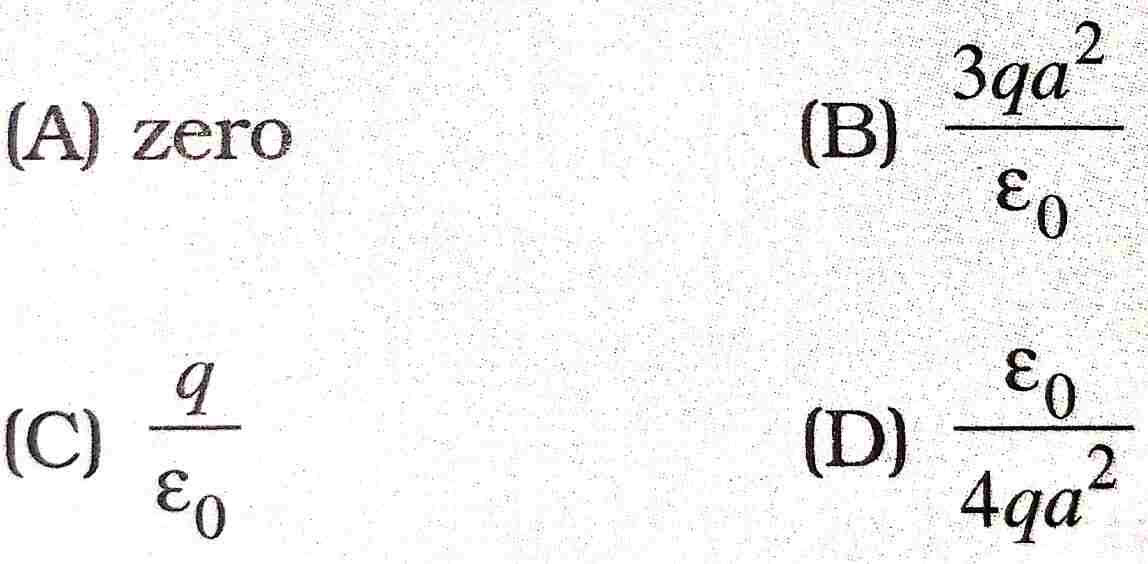37. A charge Q is placed at the mouth of a conical flask. The flux of the electric field through the flask is :